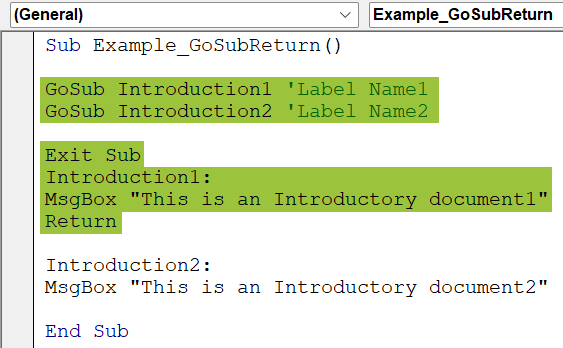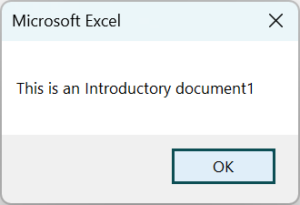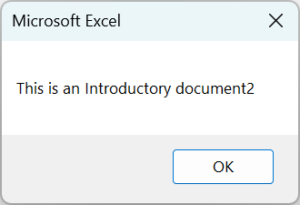# VBA Return FunctionArticle byExcelMojo Team## What is Excel VBA Return Function?

VBA Return function stops the execution of a method in which it is present and returns the control to the original method. Briefly, in VBA, if we wish to return a value to another method, we use the Return statement.

In this example, we will multiply integers using GoSub and Return statements. Write the below VBA code. Here, we take two integers as input, display their product in the Multiplication label, and then return the control using the Return statement.

Run the code, and you will be prompted to enter the numbers. Enter them in the VBA input boxes and see the result as shown below. Press OK.

###### Key Takeaways
• VBA Return Function is used after the execution of code is completed to return the control to the original procedure.
• GoSub and Return statements are used in the same sub-procedure.
• GoSub Statement is used with a user-defined function to execute the task.
• VBA Return Array from function when we declare arrays with the type of variant.
• VBA Return returns a value from any sub using a Function.

### What is GoSub Return Statement?

GoSub Return statement is used to call a subroutine within the sub-procedure. It goes to the line of code given by a label name and performs a specific task till it finds a Return statement. It is used in user-defined functions along with the Return statement.

• Syntax – GoSub Return Statement

GoSub [Labelname]
`...` line

[Labelname]:
line of code for task`...`
Return

### How to Use GoSub Return Function in VBA?

Let us now see the working of Return with GoSub Function in VBA with a simple example. Follow the below steps to understand the use of GoSub Return Statement in VBA:

1. In the Excel sheet, open Visual Basic under the Developer option and choose the Insert option to open a new module.

2. Write the below code in the VB editor as shown.:

Code:

Sub Example_GoSubReturn()
GoSub Introduction1 ‘Label Name1
GoSub Introduction2 ‘Label Name2
Exit Sub
Introduction1:
MsgBox “This is an Introductory document1”
Return
Introduction2:
MsgBox “This is an Introductory document2”
End Sub

Explanation:

GoSub Introduction1 ‘Label Name1
GoSub Introduction2 ‘Label Name2

• Commands the execution of another statement with the labels specified within the same sub-procedure.

Exit Sub
• This line exits the sub-procedure

Introduction1:
MsgBox “This is an Introductory document1.”
• This line of code is executed when we instruct the macro to go to the label Introduction1 in the Sub procedure.

Return3. Press the Run button, a green triangle in the VB ribbon, to see the result in the message box.

The first message shows the result as shown below:Press OK, and you will see another message box that shows the result below.### Examples

Let us look at some examples to understand VBA Return in detail.

#### Example #1

For Example, let us find out the volume of a cone. Here, the VBA Return function returns the value of the volume of the cone.

• Step 1: Write the following code.

Code:

Function ConeVolume(ByVal Radius As Double, ByVal Height As Double) As Double
‘Pir^2h/3
Dim Volume As Double
Volume = WorksheetFunction.Pi * Radius ^ 2 * Height / 3
ConeVolume = Volume
End Function

Dim Height As Double
Height = InputBox(“Please enter the Height of the cone”)
MsgBox “The volume of your cone is : ” & ConeVolume(Radius, Height)
Return
End Sub

Explanation:

Function ConeVolume(ByVal Radius As Double, ByVal Height As Double) As Double

• Here, the function is used, and the variants are used as Double data type.

• This sub procedure is used to get the numbers of radius and height parameters.

• For radius, we will see the Input box with this assigned message and enter the radius value there.

MsgBox “The volume of your cone is : ” & ConeVolume(Radius, Height)
Return

• The message box prints the volume, and the execution is returned to the main code.
• Step 2: Run the code either by clicking on Run in the VBA ribbon or by directly using the F8 key, and we will see the first input message box for radius. Write the value of the radius and click OK, as shown below:
• Step 3: Another input box of height will appear, write the value, and click OK as shown below:
• Step 4: Finally, the Return statement will give the value for the volume of the cone as shown below:

#### Example #2

Let’s see another example of a GoSub statement with a Return for the Division of two integers.

• Step 1: Write the below code:

Code:

Sub Example2_ReturnVBA()
Dim Num
Num = InputBox(“Enter a number to be divided by 5.”)
If Num > 0 Then GoSub MyRoutine
Debug.Print Num
Exit Sub
MyRoutine:
Num = Num / 5
MsgBox Num
Return
End Sub

Explanation:

Num = InputBox(“Enter a number to be divided by 5”)

• This line is used to get an input through the input box and assign it to a variable.

If Num > 0 Then GoSub Division

• We are placing a condition; if the number is > 0, go to the label, Division.

Debug.Print Num

• This line is used to print the result.

Return

• It returns the control to the original statement.
• Step 2: Run the code and see the result as shown below:
• Step 3: Press OK. You get the result as shown below.

### Important Things to Note

• In the case of user-defined functions, the values are returned using the Return statement.
• The return statement is placed after the execution of a code line so that it will return the control to the original statement.
• In the case of the GoSub statement, both the GoSub and Return statement should be in the same procedure.
• A colon should always follow the label name in the Return statement (:)
• We can use more than one Return statement in the same code.
• We must always use Exit Sub after writing all the lines of Return statements to avoid an error message.

1. Can a VBA function return an array?

The VBA function can return arrays of any data type.

2. How do I insert a carriage return in Excel VBA?

In VBA, there are three different constants used to add a line break/carriage Return:
1) vbNewLine: It inserts a newline character that enters a line.
2) vbCrLf: this constant stands for Carriage Return and Line feed. Here, Cr brings the cursor to the start of the line, and Lf gets the cursor down to the following line.
3) vbLf: This constant stands for line feed character. When we use this constant within two strings, it returns a line feed character, adding a new line for the second string.

3. How do I remove a carriage return in VBA?

There are three simple ways to remove a carriage return in VBA:
1) Remove carriage Returns using Find and Replace
2) Use of Excel functions such as SUBSTITUTE or CHAR to Remove Carriage Returns.
The SUBSTITUTE function replaces existing text with new text within a text string.
The CHAR function returns the character specified by the code number from the set of characters in your computer.
3) Erase carriage Returns using VBA in Excel: Write a code in VBA to remove carriage Return and Run the code.

4. What does not return value in VBA?

A sub does not return a value in VBA. Only a function does.

4. How do I return a variable value in VBA?

To return a variable value in VBA
Place the Return statement at the point where the execution of the task is completed.
Track the Return keyword using an expression that produces the value we want to return to the calling code.
We can have more than one Return statement in the same procedure.
Thus, you can place the VBA Return value from sub.

This has been a guide to VBA Return. Here we learn how to use GoSub Return statement in Excel VBA, along with examples & downloadable excel template. You can learn more from the following articles –